Next: Probabilistic Forms Up: Image Generators Previous: Image Generators

### Example

Consider a toy example in 1D, as shown in figure 1. In the example there are two shapes,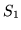and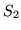, each of different size (length) which results in the generation of four different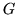vectors: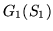to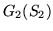. Note that at the interface between the shapes, the values of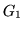are between 0.0 and 1.0, representing the partial volume fraction. The transformation,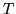, effects both the global position (and stretching) of the shapes in the image as well as the partial volume fractions.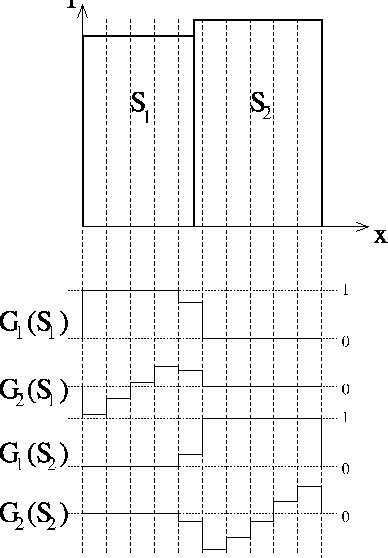Next: Probabilistic Forms Up: Image Generators Previous: Image Generators